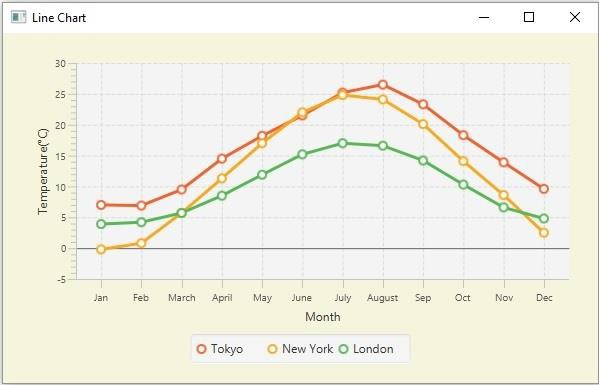# JavaFX line chart example with multiple series (lines)

Inline chart, the data values have represented a series of points connected by a line. In JavaFX, you can create a line chart by instantiating the javafx.scene.chart.LineChart class.

While instantiating this class you must pass the two objects of the Axis class representing the x and y-axis (as parameters of the constructor). Since the Axis class is abstract you need to pass objects of its concrete subclasses, NumberAxis (for numerical values) or, CategoryAxis (String values).

## Line chart with multiple series

• The XYChart.Data class represents a data point in the chart, you can create a data point by instantiating this class.

XYChart.Data dataPoint1 = new XYChart.Data(x-value, y-value)
XYChart.Data dataPoint2 = new XYChart.Data(x-value, y-value)
XYChart.Data dataPoint3 = new XYChart.Data(x-value, y-value)
• Once you create all the required data points, you can create a series you need to instantiate the XYChart.Series class and add the data points to it.

XYChart.Series series = XYChart.Series
series.getData().add(dataPoint3);
• You can create as many numbers of such series as required.

## Example

The following example demonstrates the creation of a line chart with multiple lines.

import javafx.application.Application;
import javafx.scene.Scene;
import javafx.stage.Stage;
import javafx.scene.chart.CategoryAxis;
import javafx.scene.chart.LineChart;
import javafx.scene.chart.NumberAxis;
import javafx.scene.chart.XYChart;
import javafx.scene.layout.StackPane;
public class LineChart_MultipleLines extends Application {
public void start(Stage stage) {
//Defining the x an y axes
CategoryAxis xAxis = new CategoryAxis();
NumberAxis yAxis = new NumberAxis();
//Setting labels for the axes
xAxis.setLabel("Month");
yAxis.setLabel("Temperature(°C)");
//Creating a line chart
LineChart<String, Number> linechart = new LineChart<String, Number>(xAxis, yAxis);
//Preparing the data points for the line1
XYChart.Series series1 = new XYChart.Series();
//Preparing the data points for the line2
XYChart.Series series2 = new XYChart.Series();
//Preparing the data points for the line3
XYChart.Series series3 = new XYChart.Series();
//Setting the name to the line (series)
series1.setName("Tokyo");
series2.setName("New York");
series3.setName("London");
//Setting the data to Line chart
//Creating a stack pane to hold the chart
StackPane pane = new StackPane(linechart);
pane.setStyle("-fx-background-color: BEIGE");
//Setting the Scene
Scene scene = new Scene(pane, 595, 350);
stage.setTitle("Line Chart");
stage.setScene(scene);
stage.show();
}
public static void main(String args[]){
launch(args);
}
}

## Output